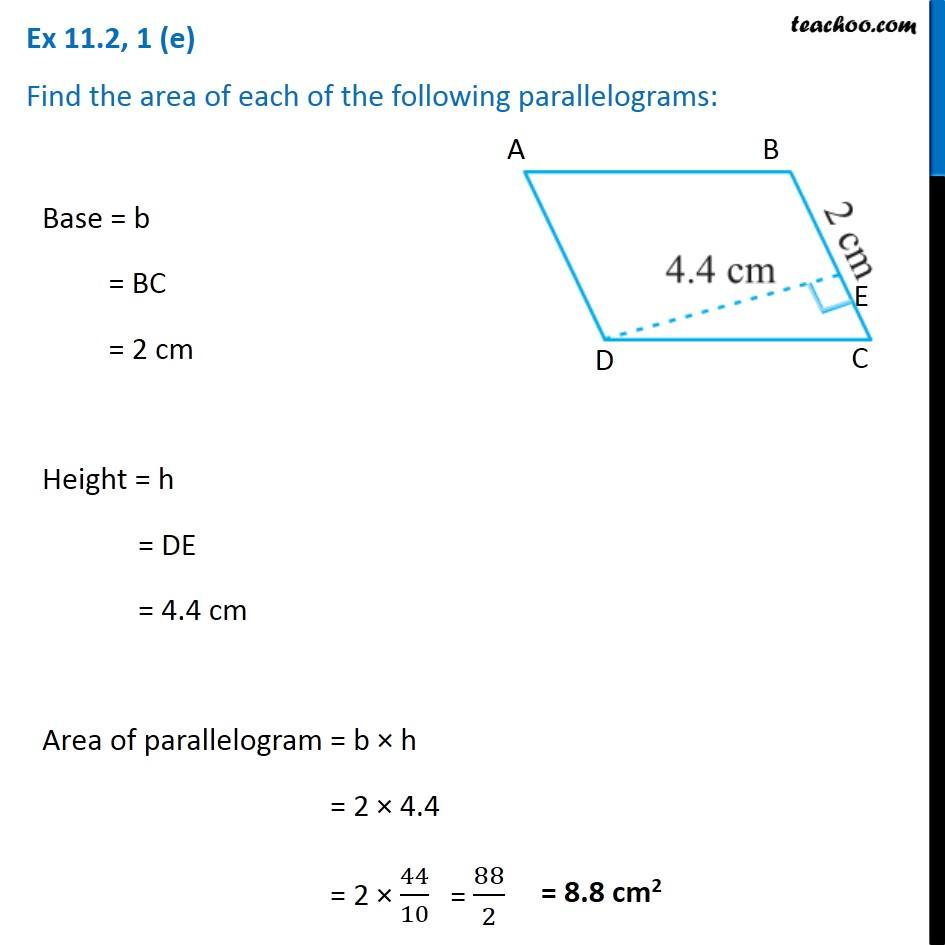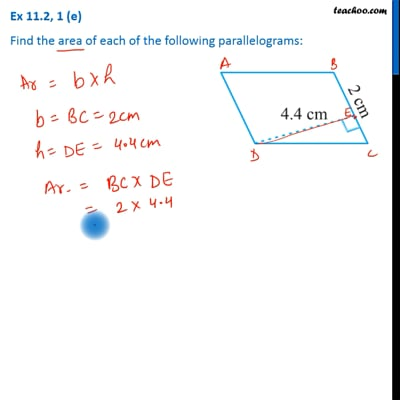Ex 11.2

Chapter 11 Class 7 Perimeter and Area
Serial order wiseThis video is only available for Teachoo black users

Get live Maths 1-on-1 Classs - Class 6 to 12

### Transcript

Ex 11.2, 1 (e) Find the area of each of the following parallelograms: Base = b = BC = 2 cm Height = h = DE = 4.4 cm Area of parallelogram = b × h = 2 × 4.4 = 2 × 44/10 = 88/2 = 8.8 cm2